Download the eSaral app and start learning from Kota's top IITians and doctors.

# Definite Integration - JEE Main Previous Year Question with Solutions

JEE Main Previous Year Question of Math with Solutions are available at eSaral. Practicing JEE Main Previous Year Papers Questions of mathematics will help the JEE aspirants in realizing the question pattern as well as help in analyzing weak & strong areas. eSaral helps the students in clearing and understanding each topic in a better way. eSaral is providing complete chapter-wise notes of Class 11th and 12th both for all subjects. Besides this, eSaral also offers NCERT Solutions, Previous year questions for JEE Main and Advance, Practice questions, Test Series for JEE Main, JEE Advanced and NEET, Important questions of Physics, Chemistry, Math, and Biology and many more. Download eSaral app for free study material and video tutorials.
Q. $\int_{0}^{\pi}[\cot x] d x,$ where $[.]$ denotes the greatest integer function, is equal to – (1) –1 $(2)-\frac{\pi}{2}$            (3) $\frac{\pi}{2}$            (4) 1 [AIEEE-2009]
Ans. (2)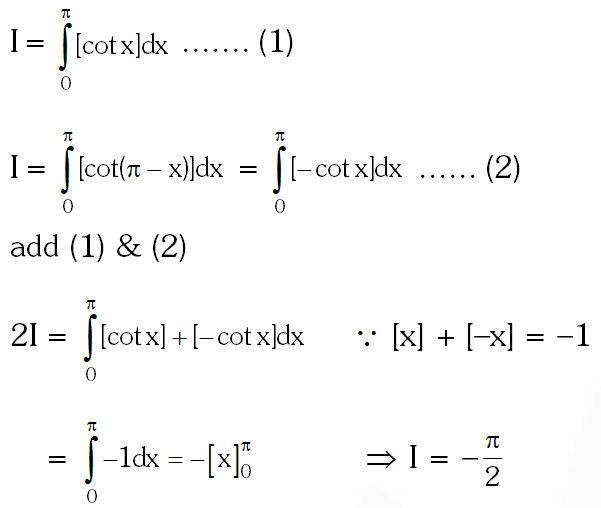Q. Let p(x) be a function defined on R such that p'(x) = p'(1 – x), for all x $\in$0, 1], p(0) = 1 and p(1) = 41. Then $\int_{0}^{1}$ p(x) dx equals :- (1) $\sqrt{41}$ (2) 21 (3) 41 (4) 42 [AIEEE-2010]
Ans. (2)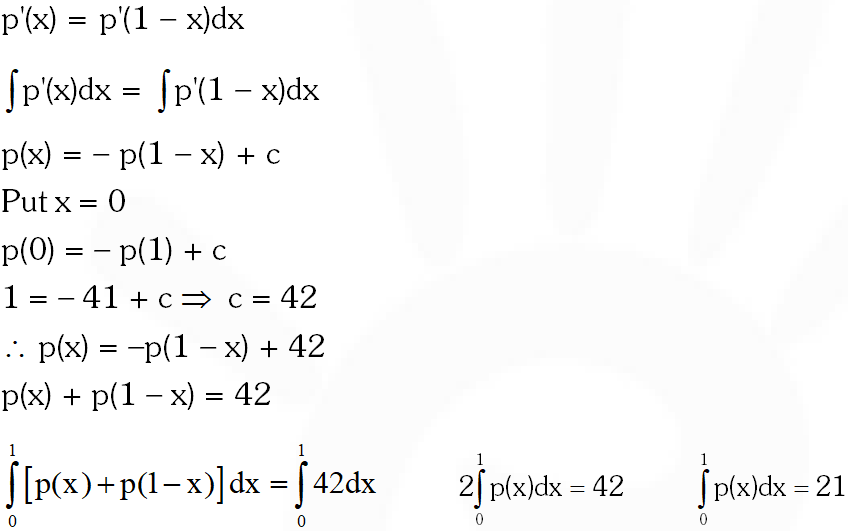Q. The value of $\int_{0}^{1} \frac{8 \log (1+x)}{1+x^{2}} d x$ is :- (1) $\frac{\pi}{2} \log 2$ (2) $\log 2$ (3) $\pi \log 2$ (4) $\frac{\pi}{8} \log 2$ [AIEEE-2011]
Ans. (3)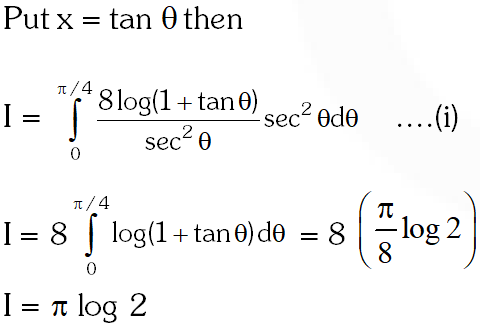Q. Let [.] denote the greatest integet function then the value of $\int_{0}^{1.5} \mathrm{x}\left[\mathrm{x}^{2}\right] \mathrm{dx}$ is :- ( 1)$\frac{5}{4}$ (2) 0 (3) $\frac{3}{2}$ (4) $\frac{3}{4}$ [AIEEE-2011]
Ans. (4)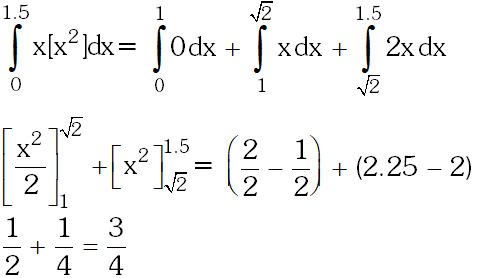Q. If $\mathrm{g}(\mathrm{x})=\int_{0}^{\mathrm{x}} \cos 4 \mathrm{t} \mathrm{dt},$ then $\mathrm{g}(\mathrm{x}+\pi)$ equals : (1) $\mathrm{g}(\mathrm{X}) \cdot \mathrm{g}(\pi)$ (2) $\frac{\mathrm{g}(\mathrm{x})}{\mathrm{g}(\pi)}$ (3) $\mathrm{g}(\mathrm{x})+\mathrm{g}(\pi)$ (4) $\mathrm{g}(\mathrm{x})-\mathrm{g}(\pi)$ [AIEEE-2012]
Ans. (3,4)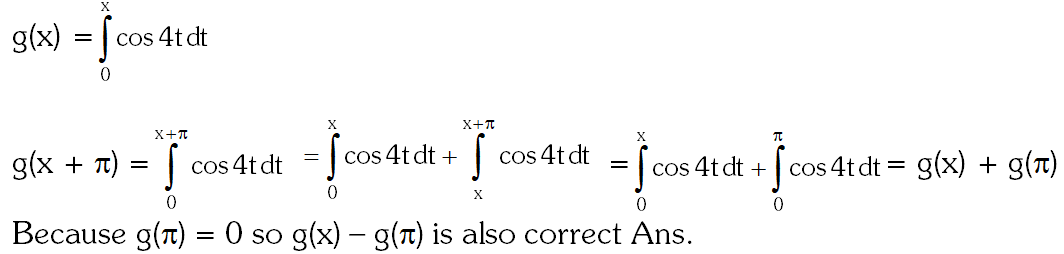Q. Statement-I : The value of the integral $\int_{\pi / 6}^{\pi / 3} \frac{\mathrm{dx}}{1+\sqrt{\tan \mathrm{x}}}$ is equal to $\frac{\pi}{6}$ Statement-II : $\int_{a}^{b} f(x) d x-\int_{a}^{b} f(a+b-x) d x$ (1) Statement-I is true, Statement-II is true; Statement-II is a correct explanation for Statement- I. (2) Statement-I is true, Statement-II is true; Statement-II is not a correct explanation for Statement-I. (3) Statement-I is true, Statement-II is false. (4) Statement-I is false, Statement-II is true. [JEE-MAIN-2013]
Ans. (4)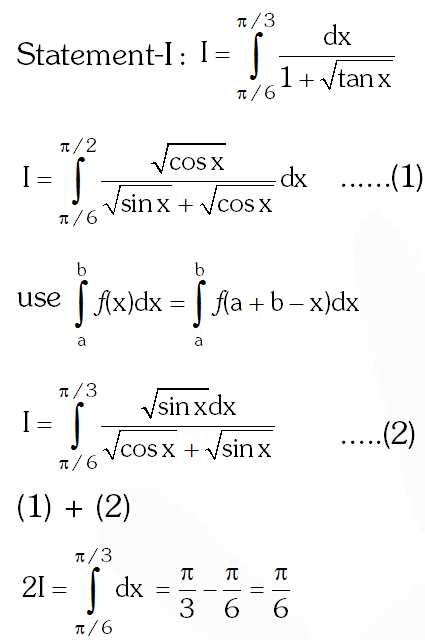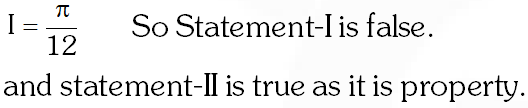Q. The integral $\int_{0}^{\pi} \sqrt{1+4 \sin ^{2} \frac{x}{2}-4 \sin \frac{x}{2}} d x$ equals : (1) $\pi-4$ (2) $\frac{2 \pi}{3}-4-4 \sqrt{3}$ (3) $4 \sqrt{3}-4$ (4) $4 \sqrt{3}-4-\frac{\pi}{3}$ [JEE-MAIN-2014]
Ans. (4)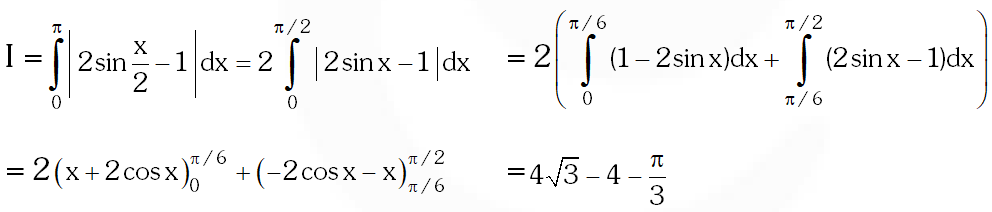Q. The integral $\int_{2}^{4} \frac{\log x^{2}}{\log x^{2}+\log \left(36-12 x+x^{2}\right)} d x$ is equal to : (1) 1 (2) 6 (3) 2 (4) 4 [JEE-MAIN-2015]
Ans. (1)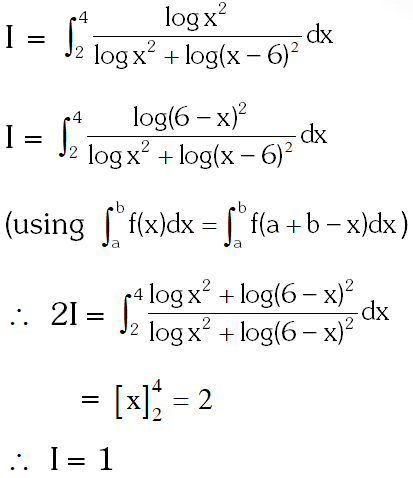Q. The integral $\int_{\frac{\pi}{4}}^{\frac{3 \pi}{4}} \frac{\mathrm{dx}}{1+\cos x}$ is equal to :- (1) –1 (2) –2 (3) 2 (4) 4 [JEE-MAIN-2017]
Ans. (3)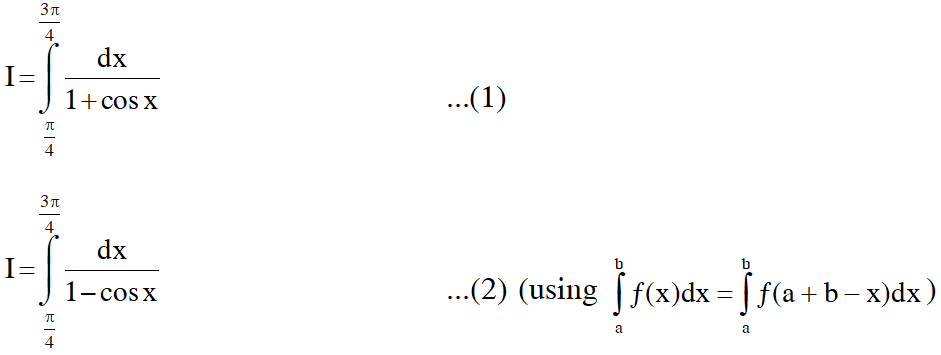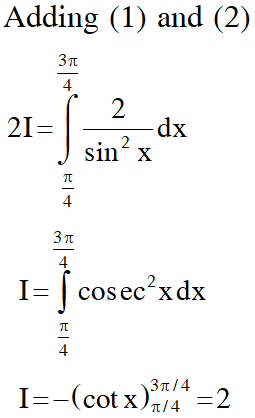Q. The value of $\int_{-\frac{\pi}{2}}^{\frac{\pi}{2}} \frac{\sin ^{2} x}{1+2^{x}} d x$ is : ( 1)$\frac{\pi}{2}$ (2) $4 \pi$ (3) $\frac{\pi}{4}$ (4) $\frac{\pi}{8}$ [JEE-MAIN-2018]
Ans. (3)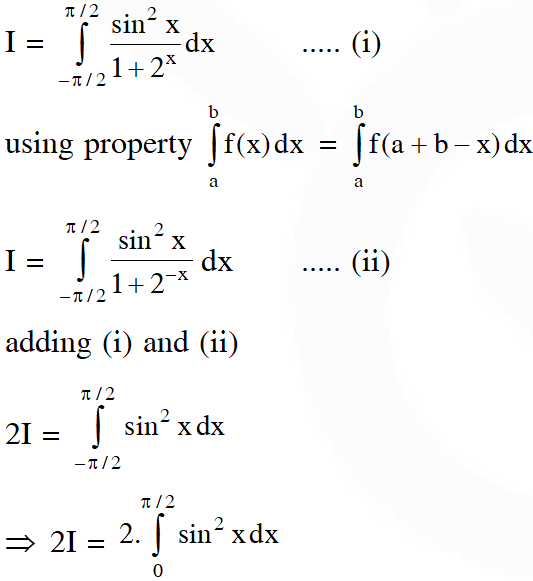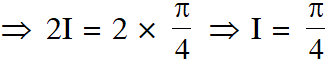guna sekhar sai
March 4, 2021, 10:51 a.m.
these questions are not enough for practice please increase the number of questions
Tushar Sahu
Dec. 24, 2020, 6:46 p.m.
If there will be more questions Than it will be much better So increase the number of questions 😁😁🤘 Thanks to eSaral
Niharika
Nov. 22, 2020, 12:18 p.m.
Want more problems
bhuvan kumar
Sept. 23, 2020, 10:17 a.m.
sir can you put some latest years bits
nandyy
Sept. 15, 2020, 6:19 p.m.
IT IS USE FULL
nandyy
Sept. 15, 2020, 6:14 p.m.
it is very usefull \
Kavya
Sept. 13, 2020, 8:19 a.m.
It is very much useful.. Thank you
Nikh
Sept. 12, 2020, 3:22 p.m.
Superb
Manoj
Aug. 31, 2020, 12:56 p.m.
Thanks
roja
Aug. 22, 2020, 12:11 p.m.
superb
Amruta
Aug. 12, 2020, 9:50 a.m.
An excellent work done by saral...it helped me alot...